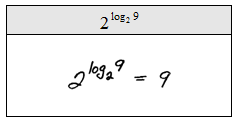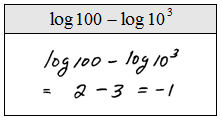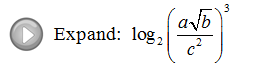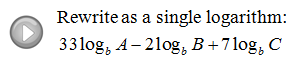## Algebra

Showing posts with label properties of the log. Show all posts
Showing posts with label properties of the log. Show all posts

### Solving Logarithmic Equations

Use the one-to-one property for logarithms to solve logarithmic equations.

If we are given an equation with a logarithm of the same base on both sides we may simply equate the arguments.

Step 1: Use the rules of exponents to isolate a logarithmic expression (with the same base) on both sides of the equation.
Step 2: Set the arguments equal to each other.
Step 3: Solve the resulting equation.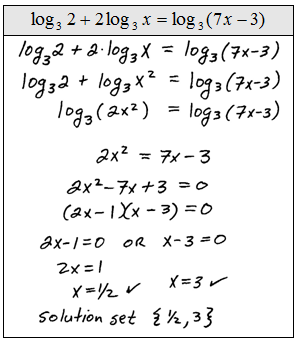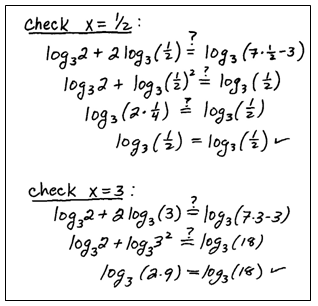Be sure to check to see if the solutions that you obtain solve the original logarithmic equation. In this study guide we will put a check mark next to the solution after we determine that it really does solve the equation. This process sometimes results in extraneous solutions so we must check our answers.
Solve.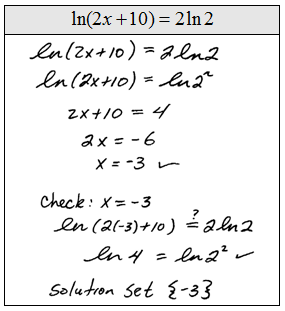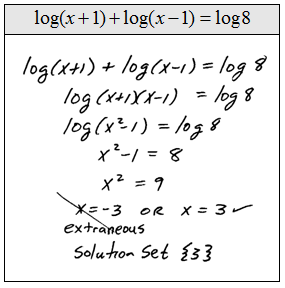Of course, equations like these are very special.  Most of the problems that we will encounter will not have a logarithm on both sides. The steps for solving them follow.

Step 1: Use the properties of the logarithm to isolate the log on one side.
Step 2: Apply the definition of the logarithm and rewrite it as an exponential equation.
Step 3: Solve the resulting equation.If the answer to the logarithmic equation makes the argument negative then it is extraneous.  This does not preclude negative answers. We must be sure to check all of our solutions.

Instructional Video: Solving Logarithmic Equations

Solve.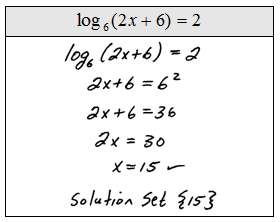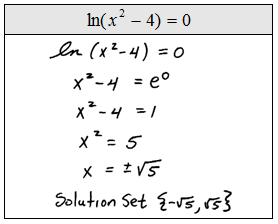Tip:  Not all negative solutions are extraneous!  Look at the previous set of problems and see that some have negative answers. The check mark indicates that we actually plugged the answers in to see that they do indeed solve the original.  Please do not skip this step, extraneous solutions occur often.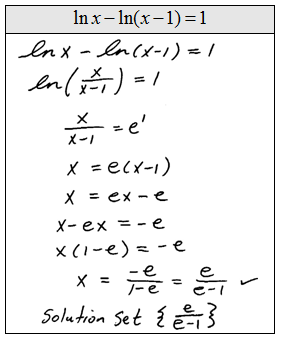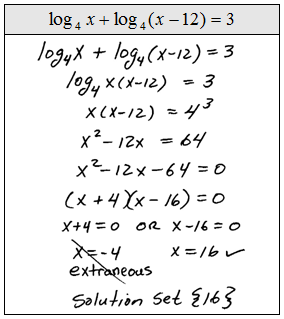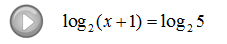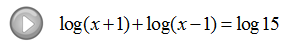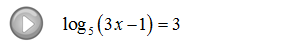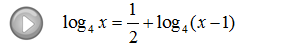### Properties of the Logarithm

The following properties of the logarithm are derived from the rules of exponents.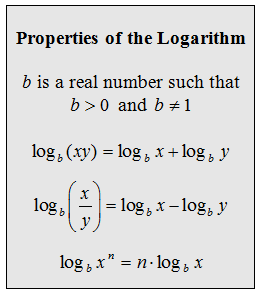The properties that follow below are derived from the fact that the logarithm is defined as the inverse of the corresponding exponential.To familiarize ourselves with the properties we will first expand the following logarithms. (Assume all variables are positive.)

Expand.Notice that there is no explicit property that allows us to work with nth roots within the argument of the logarithm.  To simplify these, first change the root to a rational exponent then apply the power rule.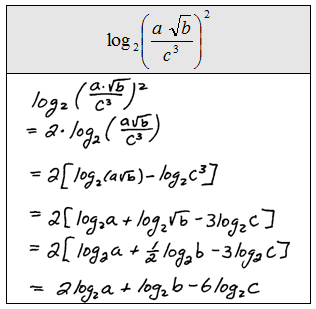When expanding, notice that we must use the same base throughout the expression. For the next set of problems we will first use the properties to expand then substitute in the appropriate values as the last step.
Evaluate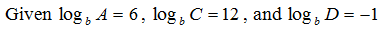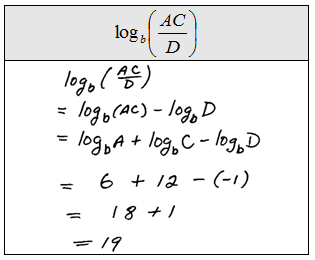Expanding is useful for learning the rules and properties associated with logarithms but as it turns out, in practice, condensing down to a single logarithm is the skill that we really need to focus on.

Rewrite as a single logarithm (condense).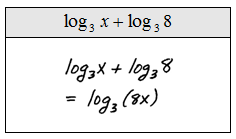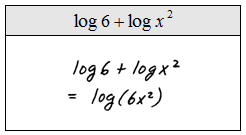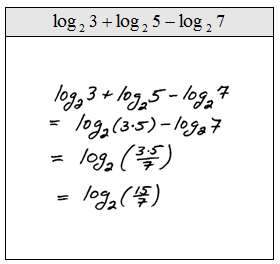Tip: When simplifying these down to one logarithm use only one operation at a time and work from left to right. Combining or skipping steps usually leads to mistakes. Do not race, work slowly, and pay attention to notation.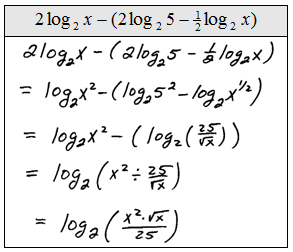Evaluate (Round to the nearest ten thousandths where appropriate).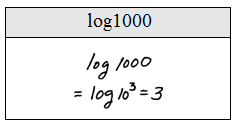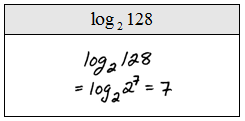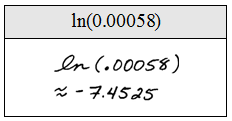Simplify.